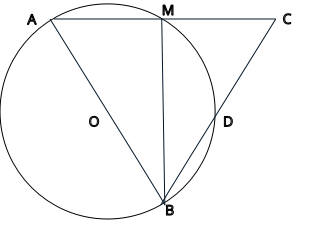### Prove: △AMB ≅ △CMBClick or touch to draw on the diagram
Circle O with diameter AB, arc AM = arc DM
arc AB = 180
∠AMB = 90
ASA (Angle-Side-Angle)
△AMB ≅ △CMB
Given
∠ABM = (1/2) * arc AM and ∠CBM = (1/2) * arc DM
Inscribed angle measures half its intercepted arc
∠CMB = 90
Substitution
Substitution
Inscribed angle measures half its intercepted arc
Reflexive
∠ABM = ∠CBM
The angles in a linear pair are supplementary
MB = MB
∠AMB = (1/2) * arc AB
Definition